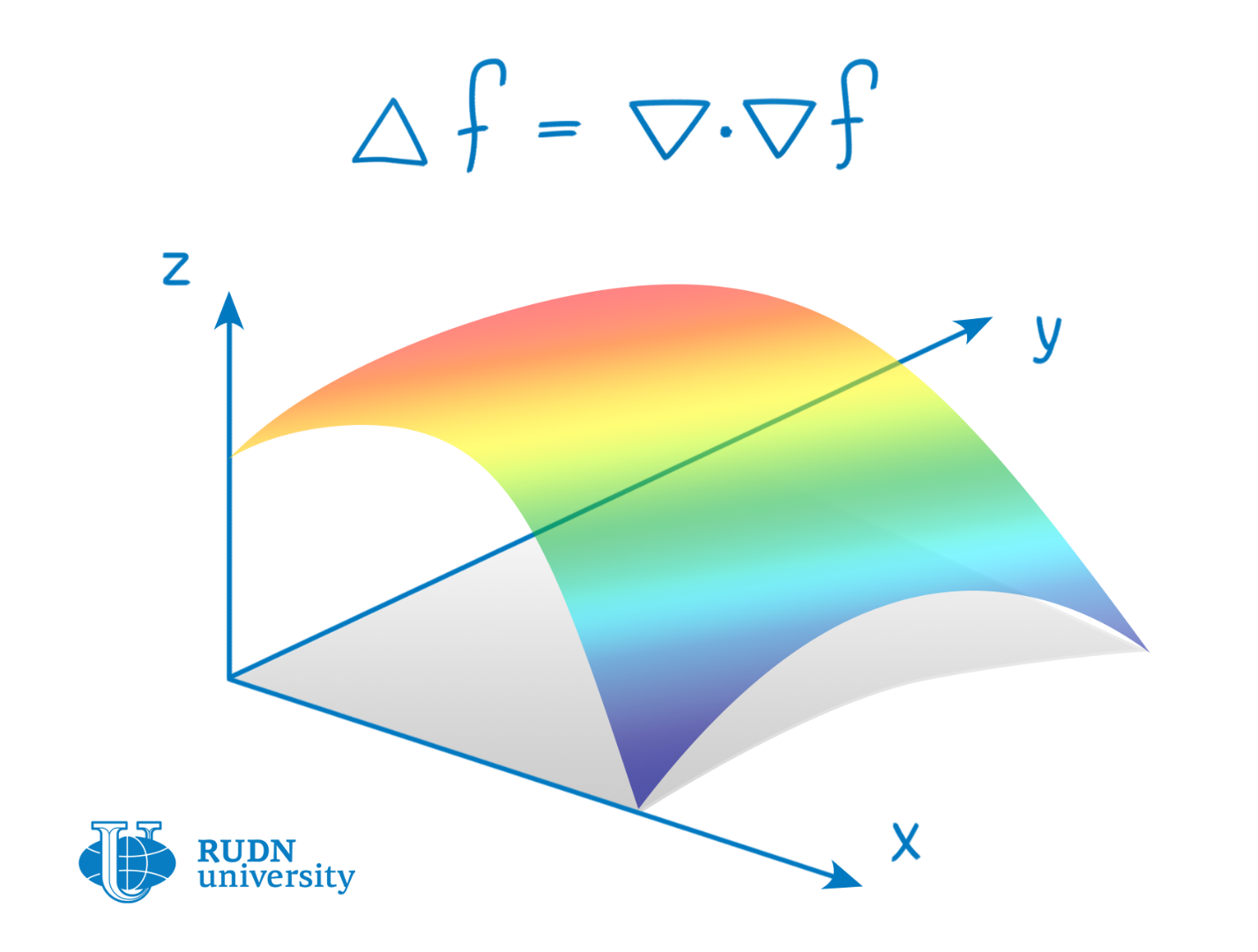RUDN University mathematicians have obtained an estimate of inverse problems of the Laplace differential equation, which allows describing the type of space under study

# RUDN University mathematicians have obtained an estimate of inverse problems of the Laplace differential equation, which allows describing the type of space under study

RUDN mathematicians received an estimate of the solution of inverse problems of the Laplace differential equation. Having a set of values that characterize the Laplace map and the Dirichlet-Neumann limit parameters, they were able to obtain conditions that determine the type of space under study. The obtained result allows us to describe the nature of physical space in the problems of electrostatics, electrodynamics, quantum mechanics, continuum physics and in the problem of studying the thermal conductivity of the medium.RUDN mathematicians received an estimate of the solution of inverse problems of the Laplace differential equation. Having a set of values that characterize the Laplace map and the Dirichlet-Neumann limit parameters, they were able to obtain conditions that determine the type of space under study. The obtained result allows us to describe the nature of physical space in the problems of electrostatics, electrodynamics, quantum mechanics, continuum physics and in the problem of studying the thermal conductivity of the medium.

When solving physical problems using mathematical methods, functions are put in accordance with certain physical processes. For example, to describe the thermal conductivity process, we need the Laplace differential equation-the Laplace map. These functions operate within a specific characteristic space that corresponds to the physical model of the phenomenon in question.

Experimental data of a physical problem can be a set of values that characterize the type of Laplace mapping for a specific space. Direct problems of the differential equation allow us to obtain this set of characteristic values in the known mathematical form of the Laplace map. In this case, the solution of inverse problems of these equations for a known set of characteristic values allows us to study the mathematical form of space, and therefore the physical process of the problem under study.

The space is characterized by a metric-a rule for distributing elements in this space. Knowing the mathematical form of the space metric, you can get the space structure of the physical model of the problem under study. Thus, by analyzing neural activity data (characteristic values), you can get a metric of the neural network space that will be associated with the physical model of this network.

It is known that the type of metric can be uniquely defined up to the level at which two metrics ― g1 and g2 ― become equivalent for a slightly different set of characteristic values, so the solution will not be the only one. However, if there are two metrics with the same set of characteristic values, a conversion is allowed that converts one metric to another.

For an adequate description of the type of space under study, it is necessary to make sure that the found metric type is correct when solving a direct problem of differential equations. For such verification, a tool can be used to study the uniqueness of the solution of inverse problems on an arbitrarily bounded area of space.

After considering a given set of characteristic values on an arbitrarily fixed domain, the authors obtained a mathematical estimation of the uniqueness of the solution for the inverse problem in determining the metric of the Laplace operator.

Another type of input for the inverse problem can be boundary conditions under which the behavior of the physical model is known. In this case, the authors used data on Dirichlet-Neumann boundary conditions for Laplace mapping on an arbitrary region of the studied space to obtain an estimate of the uniqueness of the solution of the inverse problem.

Article in the journal Inverse Problems & Imaging.

International Projects View all
15 Dec 2018
533
30 Jan 2018
The conference on international arbitration, where law students from European universities simulate court proceedings and alternately defend the interests of the respondent and the orator.
538
30 Dec
Biologists from RUDN University discovered the secret of flaxseed oil with long shelf life

Biologists from RUDN University working together with their colleagues from the Institute of Molecular Biology of the Russian Academy of Sciences and the Institute of Flax studied the genes that determine the fatty acid composition in flaxseed oil and identified polymorphisms in six of them. The team also found out what gene variations could extend the shelf life of flaxseed oil. This data can be used to improve the genetic selection of new flax breeds. The results were published in the BMC Plant Biology journal.

133
19 Apr
Dentists from RUDN University Presented a New Classification of Root Canal Shape Changes

Individual characteristics of the shape and cross-section of the root canal are one of the main issues for dentists. When treating a root canal, a doctor needs to properly clean it, fill it, and carry out a rebuilding procedure so that a canal is sealed. The first stage of endodontic treatment requires detailed knowledge of root canal anatomy. A team of dentists from RUDN University studied and classified various changes in root canal shapes. The new classification will help doctors avoid diagnostic errors, better select their tools, and treat patients more efficiently.

30
19 Apr
A chemist from RUDN developed a green catalyst for pharmaceutical and industrial chemistr

Many production facilities (e.g. plastic manufacturers, pharma companies, and others) use nanocatalysts that contain palladium—an expensive component that is not sustainably produced. A chemist from RUDN University found a way to reduce palladium consumption and to make its manufacture more eco-friendly. He developed a catalyst based on a substance that comes from plant waste. Using his invention, manufacturers could cut palladium consumption in half. Moreover, new catalysts can be reused multiple times without any decrease in efficiency.

29